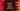# Python program to convert a binary to decimal## Python program to convert a binary to decimal:

Binary number is represented in two digits 0 and 1. Binary number system is a base-2 number system. Similarly, decimal is a base 10 number system. Each number is represented by 0, 1, 2, 3, 4, 5 , 6, 7, 8 and 9.

We can convert a binary number to decimal easily. There is an algorithm that we need to use or we can use the inbuilt int method to do the conversion.

In this post, I will show you different ways to do binary to decimal conversion in Python.

### How to convert a binary value to decimal:

To convert a binary value to decimal, we need to multiply each digit of the binary number with the power of 2 and sum the results to find the decimal.

For example, let’s take a look at the binary value 10111.

We can convert it to a decimal number as like below:

``1*2^4 + 0*2^3 + 1*2^2 + 1*2^1 + 1*2^0``

It is 23.

Let me show you different ways to convert a binary to decimal in Python:

### Example 1: Convert binary integer to decimal:

Let’s keep the binary value in an integer variable and convert it to decimal. We will take the number as input from the user.

``````binary = int(input("Enter the binary number: "))

decimal = 0
multiplier = 1

while binary > 0:
last_digit = binary % 10
decimal = decimal + (last_digit * multiplier)
multiplier = multiplier * 2
binary = int(binary/10)

print(f'Decimal value: {decimal}')``````

Here, -decimal is to hold the decimal value. It is asking the binary value as input from the user and storing that value in the binary variable.

• multiplier is the multiplier to multiply with the digit.
• The while loop will run until the value of binary is 0.

• Get the last digit and store it in last_digit.
• Multiply the last digit with the multiplier and add it to the decimal value.
• Update the value of multiplier i.e. multiply the multiplier value with 2.
• Remove the last digit from the binary and assign it to binary.
• At the end, print the decimal value.

### Example 2: Convert binary string to integer:

We can also convert a binary string to an integer. For that, we have to iterate through the characters of the string one by one.

First of all, we need to reverse the string and iterate through the characters of the string. We are traversing through the characters of the string from end to start.

Below is the complete program:

``````binary = input("Enter the binary number: ")

decimal = 0
multiplier = 1

for d in binary[::-1]:
last_digit = int(d)
decimal = decimal + (last_digit * multiplier)
multiplier = multiplier * 2

print(f'Decimal value: {decimal}')``````

binary[::-1] reverses the string and the for loop is iterating through the characters of the reverse string.

If you run this program, it will give similar output.

### Example 3: Convert binary to decimal recursively:

We can also convert a binary value to decimal recursively. A recursive function calls the same function again and again until it gets a final result.

Below program uses a recursive function to find the decimal value for a binary:

``````def binaryToDecimal(num, multiplier):
if num == 0:
return 0
else:
last_digit = num % 10
num = int(num/10)
last_digit = last_digit * multiplier
return last_digit + binaryToDecimal(num, multiplier*2)

binary = int(input("Enter the binary number: "))

print(f'Decimal value: {binaryToDecimal(binary, 1)}')``````
• binaryToDecimal is the recursive function. It calls itself again and again recursively until it gets the final result.
• Each time, we are removing the rightmost digit from the binary number and call the same method again.
• It will run till all the digits from the number are removed.

If you run the program, it will give a similar result.

### Example 4: Python way to convert binary to decimal:

Python int method provides a way to convert a binary value to decimal. This method is defined as like below:

``int(n, base)``

It converts a given number n to decimal. n is the number to convert and base is the base of the n.

We will pass the binary value as string and pass the second parameter as 2. It will return the decimal value.

Let’s take a look at the program:

``````binary = input("Enter the binary number: ")

print(f'Decimal value: {int(binary, 2)}')``````

It will convert the binary to decimal and print the result.

If you want the result in float, you need to wrap the result in float():

``````binary = input("Enter the binary number: ")

print(f'Decimal value: {float(int(binary, 2))}')``````

It will print the decimal value in float:

``````Enter the binary number: 10111
Decimal value: 23.0``````